Click to Chat

1800-1023-196

+91-120-4616500

CART 0

• 0

MY CART (5)

Use Coupon: CART20 and get 20% off on all online Study Material

ITEM
DETAILS
MRP
DISCOUNT
FINAL PRICE
Total Price: Rs.

There are no items in this cart.
Continue ShoppingAtoms and Molecules

What is atom?Atom is the smallest particle of matter that can take part in a chemical reaction.

Atom is not capable of independent existence.

Two or more atoms combine together to form molecules.

Dalton’s Atomic Theory

By observing the laws of chemical combination discussed above, John Dalton (1808) proposed atomic theory of matter. The main points of Dalton’s atomic theory are as follows:

Matter is made up of extremely small, indivisible particles called atoms.

Atom of same substance are identical in all respect i.e. they posses same size, shape, mass, chemical properties etc.

Atom of different substances are different in all respect i.e. they posses different shape, size, mass and chemical properties etc.

Atom is the smallest particle that takes part in chemical reactions.

Atom of different elements may combine with each other in a fixed, simple, whole number ratio to form compound atoms.

Atom can neither be created nor destroyed i.e. atoms are indestructible.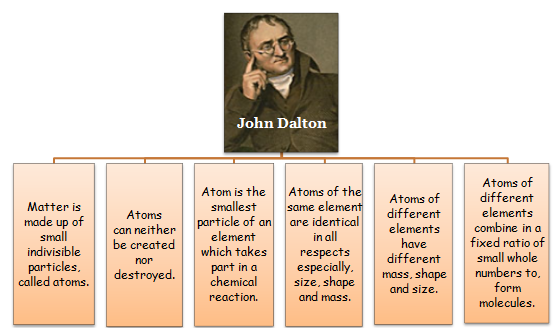Limitations of Dalton’s Atomic Theory

The main failures of Dalton’s atomic theory are:

Atom was no more indivisible. It is made up of various sub-atomic particles like electrons, proton and neutron etc.

It failed to explain how atoms of different elements differ from each other.

It failed to explain how and why atoms of elements combine with each other to form compound atoms or molecules.

It failed to explain the nature of forces that bind together different atoms in molecules.

It failed to explain Gay Lussac’s law of combining volumes.

It did not make any distinction between ultimate particle of an element that takes part in reaction (atoms) and the ultimate particle that has independent existence (molecules).

Modern Atomic theory or Modified Atomic Theory

Atom is no longer supposed to be indivisible. Atom has a complex structure and is composed of sub-atomic particles such as electrons protons and neutrons.

Atom of the same element may not be similar in all respects e.g. isotopes.

Atom of different elements may be similar in one or more respects e.g. isobars.

Atom is the smallest unit which takes part in chemical reactions.

The ratio in which atoms unite may be fixed and integral but may not be simple. e.g. In sugar molecules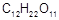, the ratio of C, H and O atoms is 12:22:11 which is not simple.

Atom of one element can be changed into atoms of other element for e.g. transmutation.

Mass of atom can be changed in energy.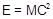according to Eienstein mass energy relationship, mass and energy are inter-convertible. Thus atom is no longer indestructible.

What is Molecule?

Molecule can be defined as the smallest particle of matter which is capable of independent existence. A molecule is assembly of two or more atoms bonded together tightly.

Molecules can be classified into two groups depending on the type the elemental atoms.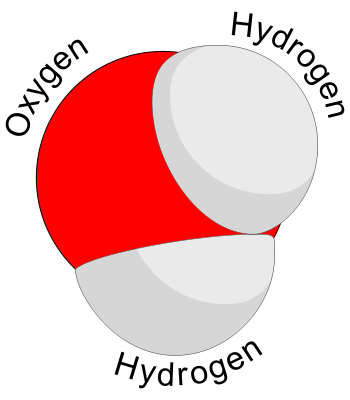Homoatomic Molecules:These  are the molecules of elements and are made up of one type of atoms only
Examples : H2, O2, N2, S8

Heteroatomic Molecules: These are the molecules of compounds and are made up of more than one type of elements.
Examples : NH3, H2O, CH4

Atomic Weight/Mass:

As atoms are very tiny particles, their absolute masses are difficult to measure. However it is possible to determine the relative masses of different atoms if small unit of mass is taken as standard (previously, this standard was mass of one atom of hydrogen and taken as unity. Later on it was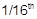part of oxygen atom and now it is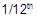part of C-12 atom).

The atomic mass of an element can be defined as the number which indicates how many times the mass of one atom of the element is heavier in comparison to the mass of one atom of hydrogen.

Atomic mass of an element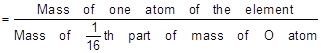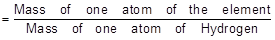Atomic  mass is not absolute but relative to the weight of the standard reference element C-12.

Atomic Mass Unit: The quantity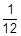mass of an atom of carbon-12 is known as the atomic mass unit and is abbreviated as amu. The actual mass of one atom of carbon-12 isorThus 1 amuAtomic mass of an elementActual mass of an elementMolecular Weight:

Molecular weight is the number of times a molecule of any compound is heavier than 1/2th of an atoms of C-12.

Molecular weight of an element =

Molecular weight is calculated by adding the atomic weights of all the atoms present in a molecule.

Example, Molecular weight of water (H2O) = 2 x Atomic Weight of H + Atomic Weight of Oxygen = 2 x 1 + 16 = 18.

Determination of Atomic Weights

Atomic Weight is determined by Dulong and Petit method.

It states that “The product of atomic weights and specific heat of an element is 6.4 approximately.”

Atomic Weight x Specific Heat = 6.4

Step 1: Atomic weight (app.) = 6.4/Specific heat

Step 2: Valency = Atomic weight / Equivalent weight

Step 3: Correct atomic weight = Equivalent weight x Valency

Average Atomic Mass

Elements are found in different isotopic forms (atoms of same elements having different atomic mass),  so, one can say that atoms of same elements which have same atomic numbers but different mass numbers are called isotopes.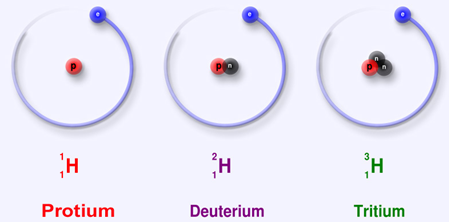In case of isotopes, atomic mass of the element is average of relative masses of different isotopes of the element.

Average atomic massExample:

There are two isotopes of chlorine Cl35 and Cl37.

Isotopes

Relative Masses

Relative Abundance (RA)

Cl35

35

3

Cl37

37

1

Atomic Mass of Chlorine = (Atomic mass of Cl35 x3) +(Atomic mass of Cl37 x1)/ (3+1) = 35.3

When atomic mass of any element is reported in grams, it is called Gram Atomic Mass( GAM)

1 GAM of Na = 23 gm of Na

1 GAM of Ca = 40 gm of Na

Similarly Carbon has two isotopes C-12 and C-13.

Refer to the following video for average atomic mass

Determination of Atomic Mass :

Applying Dulong and Petit’s law.

Cannizzaro’s methods

By mitscherlich’s law of isomorphism.

By measurement of V.D. of volatile chloride or bromide.

Dulong & Petits Law:

The product of specific heat of pure element and atomic mass of the element is equal to 6.4.

i.e. Atomic mass ´ specific heat = 6.4 (approx)

But this law is not applicable to lighter element like boron, carbon, silicon. To obtain correct atomic mass of element first of all equivalent mass of the element is known by any other method and their atomic mass = eq. weight ´ valency

In which valency has whole number value which can be deduced by dividing approximate by equivalent mass.

Dulongs and Petit’s Law: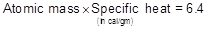Solved Example

Question: 1

The specific heat of a metal of atomic mass 32 is likely to be:

a. 0.25

b. 0.24

c. 0.20

d. 0.15

Solution:

Specific heat =Hence (C) is correct.

_______________________________________________

Question: 2

On dissolving 6 gm of metal in sulphuric acid, 13.53g of the metal sulphate was formed. The specific heat of metal is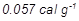. What is equivalent mass of metal, valency and exact atomic mass?

Solution:

Equivalent mass of sulphate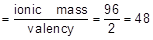Eq. weight of metal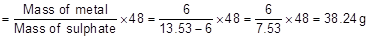Approximate atomic mass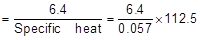ValencyExact atomic mass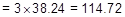Cannizzaro’s methods

If an element has several compound with other same or different elements of known atomic mass then the compound that has minimum presence of former element indicate the atomic mass of former element.

Procedure

First of all the molecular mass of all compounds known by applying V.D ´ 2 = mol. weight

By analysis the presence of the desired element in each compound is known.

The mass that is lowest among all the compound indicate the atomic mass of that element.

Solved Example

Question

Estimate the atomic mass of nitrogen given that vapour density of NH3 = 8.5, Nitrous oxide = 22, Nitric oxide = 15, Nitrogen peroxide = 23, Nitrogen

trioxide   = 38.

Solution:

Compound

V.D.

Molecular weight of compound

Nitrogen mass in compound

Least presence of nitrogen

Ammonia

8.5

17

14

So atomic mass of nitrogen = 14

Nitrous oxide

22

44

28

Nitric oxide

15

30

14

Nitrogen peroxide

23

46

14

Nitrogen trioxide

38

76

28

Law of Isomorphism

When two or more compound forms similar type of crystals or able to form mixed crystals, they are known as isomorphs. For examples: MgSO4.7H2O, ZnSO4.7H2O and FeSO4.7H2O are isomorphs of each other as their crystals posses same shape.

According to Mitscherlich [year 1819].

The valency of elements that are similarly placed to that of other elements in their isomorphs are always same.

In the above example Fe, Zn and Mg have same valency  and equal ratio of water molecule in each isomorphs.

If equivalent mass of one element is known then atomic mass can be calculated by knowing the valency of other isomorphs key element.

Solved Example

Question

Which pair of the following substances is said to be isomorphous?

a. White vitriol and blue vitriol

b. Epsom salt and Glauber’s salt

c. Blue vitriol and Glauber’s salt

d. White vitriol and Epsom salt

Solution

Epsom salt (MgSO4.7H2O) and White vitriol (ZnSO4.7H2O) contains divalent cation Mg2+ and Zn2+ and same number of water molecules as water of crystallization which hold criteria for isomorphism.

Hence (D) is correct.

Atomic mass from vapour density of a chloride:

The following steps are involved in this method

Vapour density of chloride of the element is determined

Equivalent mass of the element is determined

Let the valency of the element be x. The formula of its chloride will beMolecular weight of chloride = Atomic mass of M + 35.5 x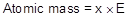So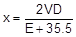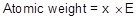Determination of Molecular Weights

Vapour density method:

Vapour Density = (Weight of certain volume of gas or vapour under certain temperature and pressure)/( Weight of the same volume of H2 under same temperature and pressure)

Molecular Weight = 2 x Vapour Density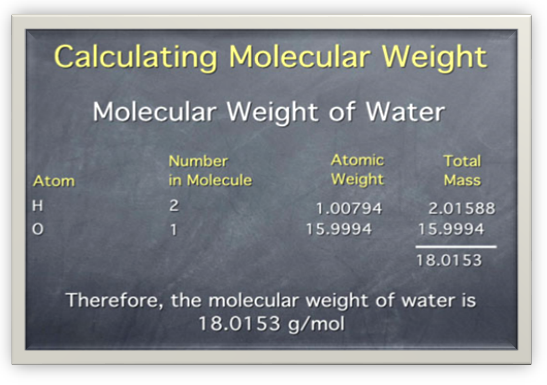Diffusion Method:

It is based on Graham’s Law of Diffusion.

The law states that “under similar conditions of temperature and pressure, the rates of diffusion of gases are inversely proportional to the square roots of their densities (or molecular weights).”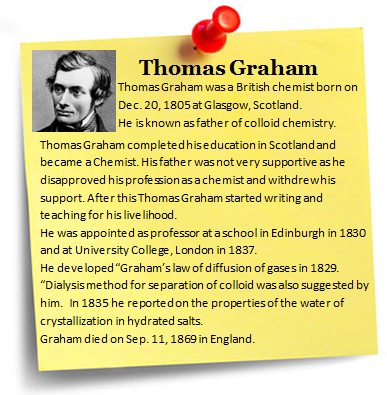The rate of diffusion (r) of a gas at constant temperature is directly preoperational to its pressure

r ∝ P at constant temperature

r ∝ 1 / √d at constant temperature

When molecular mass of a compound is expressed in grams, it is called Gram Molecular mass (GMM) or Gram Molecular weight (GMW).

Percentage Yield

Reactants, often yield quantities of products that are less than those calculated from the balanced chemical equation.

Reason behind such discrepancy may be:

Some of the reactants fail to undergo reaction due to lower amount at the end.

Some of the reactant follows other route of reaction resulting unexpected, undesired side product.

Some of the expected product recombines to form other undesired product or reverting toward reactant also known as backward reaction.

The total recovery, isolation of products is not possible due to some unavoidable reason.

So percentage yield is the ratio of actual yield (recovered) to theoretical yield multiplied by 100.Question 1: Atomic mass is the number of times an atom of any element is heavier than 1/2th of an atoms of

a.  C-12.

b.  C-13

c.  Cl -35

d. Cl -37

Question 2: What would be the molecular mass of a compound whose vapour density is 5 ?

a. 5

b. 10

c. 15

d. 20

Question 3: What is the molecular weight of HNO3?

( Given: Atomic weight of N, H and O are 14, 2 and 16 respectively)

a. 32

b. 64

c. 46

d. 23

Question 4: Isotopes of any element have

a. same atomic weight but different atomic number.

b. same atomic weight and  atomic number.

c. different atomic weight but same atomic numbers.

d. different atomic weight and atomic numbers.Q.1

Q.2

Q.3

Q.4

a

b

b

c

Related Resources

You can also refer to syllabus of chemistry for  IIT JEE

Look here for the past year papers of IIT JEE### Course Features

• 731 Video Lectures
• Revision Notes
• Previous Year Papers
• Mind Map
• Study Planner
• NCERT Solutions
• Discussion Forum
• Test paper with Video Solution